JEE  >  JEE Main Mathematics Mock - 3

# JEE Main Mathematics Mock - 3

Test Description

## 25 Questions MCQ Test JEE Main & Advanced Mock Test Series | JEE Main Mathematics Mock - 3

JEE Main Mathematics Mock - 3 for JEE 2023 is part of JEE Main & Advanced Mock Test Series preparation. The JEE Main Mathematics Mock - 3 questions and answers have been prepared according to the JEE exam syllabus.The JEE Main Mathematics Mock - 3 MCQs are made for JEE 2023 Exam. Find important definitions, questions, notes, meanings, examples, exercises, MCQs and online tests for JEE Main Mathematics Mock - 3 below.
Solutions of JEE Main Mathematics Mock - 3 questions in English are available as part of our JEE Main & Advanced Mock Test Series for JEE & JEE Main Mathematics Mock - 3 solutions in Hindi for JEE Main & Advanced Mock Test Series course. Download more important topics, notes, lectures and mock test series for JEE Exam by signing up for free. Attempt JEE Main Mathematics Mock - 3 | 25 questions in 60 minutes | Mock test for JEE preparation | Free important questions MCQ to study JEE Main & Advanced Mock Test Series for JEE Exam | Download free PDF with solutions
 1 Crore+ students have signed up on EduRev. Have you?
JEE Main Mathematics Mock - 3 - Question 1

### if the pair of lines ax2 + 2hxy + by2 + 2gx + 2fy + c = 0

Detailed Solution for JEE Main Mathematics Mock - 3 - Question 1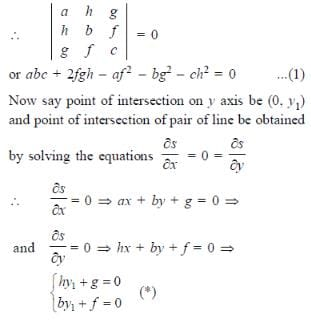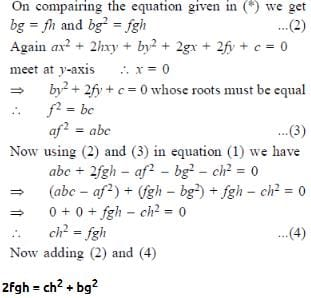JEE Main Mathematics Mock - 3 - Question 2

### The circle x2 + y2 - 8x + 4y + 4 = 0 touches

JEE Main Mathematics Mock - 3 - Question 3

### The foci of the ellipse 25(x+1)2 + 9(y+2)2 = 225 are

Detailed Solution for JEE Main Mathematics Mock - 3 - Question 3JEE Main Mathematics Mock - 3 - Question 4

The product of all roots of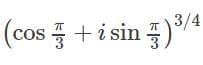is

JEE Main Mathematics Mock - 3 - Question 5

The differential equation of the family of lines passing through the origin is

Detailed Solution for JEE Main Mathematics Mock - 3 - Question 5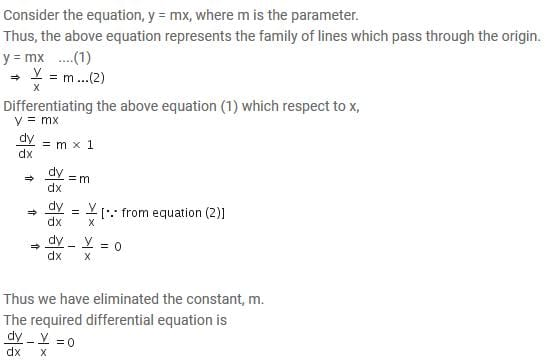JEE Main Mathematics Mock - 3 - Question 6
Log (x + y) - 2xy = 0, then y (0) =
JEE Main Mathematics Mock - 3 - Question 7

The area bounded by the curve y = x2 - 4x, x-axis and line x = 2 is

Detailed Solution for JEE Main Mathematics Mock - 3 - Question 7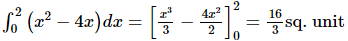JEE Main Mathematics Mock - 3 - Question 8

If f: R → R and g : R → R defined by f(x) = 2x + 3 and g(x) = x2 + 7, then the value of x for which f(g(x)) = 25 are

JEE Main Mathematics Mock - 3 - Question 9

In the following question, a Statement of Assertion (A) is given followed by a corresponding Reason (R) just below it. Read the Statements carefully and mark the correct answer-
Assertion(A): f (x)   = log x3 and g (x)   = 3 log x are equal .
Reason(R) : Two functions f and g are said to be equal if their domains, ranges are equal and f (x)   = g (x) ∀ x in the domain .

JEE Main Mathematics Mock - 3 - Question 10

Which of the following statements are true ?
(1) The amplitude of the product of complex numbers is equal to the product of their amplitudes.
(2) For any polynomial f(x) =0 with real co-efficients, imaginary roots occurs in conjugate paris.
(3) Order relation exists in complex numbers whereas it does not exist in real numbers.
(4) The value of ω used as a cube root of unity and as a fourth root of unity are different.

JEE Main Mathematics Mock - 3 - Question 11

A tangent is drawn at the point (3√3 cos θ, sin θ) 0 < θ < (π/2) of an ellipse (x2/27) + (y2/1) = 1 the least value of the sum of the intercepts on the co-ordinate axes by this tangent is attained at θ =

JEE Main Mathematics Mock - 3 - Question 12

In the following question, a Statement of Assertion (A) is given followed by a corresponding Reason (R) just below it. Read the Statements carefully and mark the correct answer-
Assertion (A):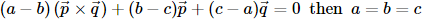Reason (R): The non zero vectors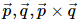are always linearly independent

JEE Main Mathematics Mock - 3 - Question 13

How many numbers between 99 and 1000 can be formed from the digits 2,3,7,0,8,6 so that in each number each digit may occur once only?

JEE Main Mathematics Mock - 3 - Question 14

The probabilities of solving a problem by three student A,B,C are 1/2, 1/3, 1/4 respectively. The probability that problem will be solved is

Detailed Solution for JEE Main Mathematics Mock - 3 - Question 14

We have, probability that A can solve the problem = P(A) = 1/2 ,
And in this way P(B) = 1/3 and P(C) = 1/4.
P(A cannot solve the problem) = 1 – P(A) = 1/2 ,
P(B cannot solve the problem) = 1 – P(B) = 1 – 1/3 = 2/3,
P(C cannot solve the problem) = 1 – P(C) = 1 – 1/4 = 3/4.
P(A, B, and C cannot solve the problem) = 1/2 x 2/3 x 3/4 = 1/4.
Therefore , P(Problem will be solve) = 1 – P(Problem is not solved by any of them)
= 1 – 1/4 = 3/4

JEE Main Mathematics Mock - 3 - Question 15

If two dice are thrown, find the probability of getting an odd number of on one and multiple of 3 on the other is

Detailed Solution for JEE Main Mathematics Mock - 3 - Question 15

Odd no. on the first die

1, 3, 5

multiple of 3 on the other die

3, 6

now let's see the combination of these two events happening simultaneously

as the question says

(1,3) , (1,6) , (3,3) , (3,6) , (5,6) , (5,3)

total no of favourable events = 6

total no of events throwing two dice simultaneously = 6² = 36

so probability = 6/36 = 1/6

JEE Main Mathematics Mock - 3 - Question 16

If the roots of ax2 + bx + c = 0 are α,β and roots of Ax2 + Bx + C = 0 are α + K, β + K, then B2 - 4AC/b2 - 4ac is equal to

JEE Main Mathematics Mock - 3 - Question 17

Let f(x) be a polynominal function of second degree,If f(1) = f(-1) and a,b,c are in A.P., then f'(a),f'(b) and f'(c) are in

JEE Main Mathematics Mock - 3 - Question 18

The total expenditure incurred by an industry under different heads is best presented as a

JEE Main Mathematics Mock - 3 - Question 19
The orthocentre of a triangle whose vertices are [(2),((√3-1)/2)], ((1/2),-(1/2)) and (2,-(1/2)) is
JEE Main Mathematics Mock - 3 - Question 20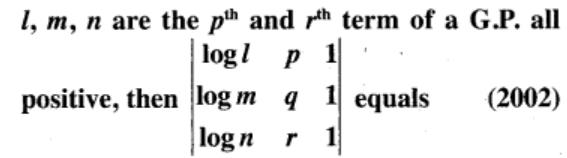Detailed Solution for JEE Main Mathematics Mock - 3 - Question 20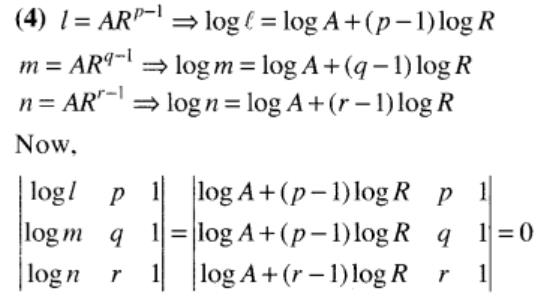*Answer can only contain numeric values
JEE Main Mathematics Mock - 3 - Question 21

A house of height 100 m subtends a right  angle at the window of an opposite house. If the height of the window be 64 m, then the distance between the two houses is

Detailed Solution for JEE Main Mathematics Mock - 3 - Question 21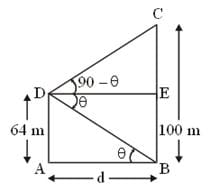*Answer can only contain numeric values
JEE Main Mathematics Mock - 3 - Question 22

The value of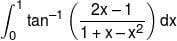is :-

Detailed Solution for JEE Main Mathematics Mock - 3 - Question 22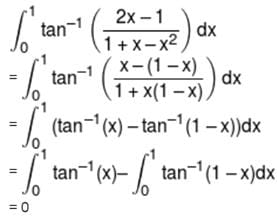*Answer can only contain numeric values
JEE Main Mathematics Mock - 3 - Question 23

The area (in sq. units) of the region A =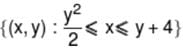is :-

*Answer can only contain numeric values
JEE Main Mathematics Mock - 3 - Question 24

A line makes α/2, β/2, γ/2 angles with positive direction of coordinate axes then cosα + cosβ + cosγ equals-

*Answer can only contain numeric values
JEE Main Mathematics Mock - 3 - Question 25

If a, b and c are perpendicular to b + c, c + a and a + b respectively and if |a + b| = 6, |b + c| = 8 and |c + a| = 10 then |a + b + c| =

## JEE Main & Advanced Mock Test Series

2 videos|325 docs|189 tests
 Use Code STAYHOME200 and get INR 200 additional OFF Use Coupon Code
Information about JEE Main Mathematics Mock - 3 Page
In this test you can find the Exam questions for JEE Main Mathematics Mock - 3 solved & explained in the simplest way possible. Besides giving Questions and answers for JEE Main Mathematics Mock - 3, EduRev gives you an ample number of Online tests for practice

## JEE Main & Advanced Mock Test Series

2 videos|325 docs|189 tests